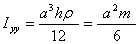EXAMPLE 2: MASS MOMENT OF INERTIACalculate the mass moment of inertia of the triangular plate about the y-axis. Assume the plate is made of a uniform material and has a mass of m.

Solution:

The mass moment of inertia about the y-axis is given by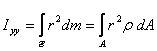The element of area in rectangular coordinate system is given by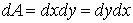The domain of the triangle is defined by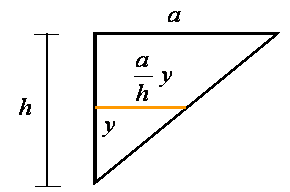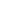The distance from the y-axis is x. Therefore, r=x. The mass moment of inertia about the y-axis can be written asFor a uniform plate the density can be calculated using the total mass and total area of the plate so thatTherefore, the moment of inertia in terms of the total mass of the cone can be written as